Butt Weld Under Axial And Transverse Loading Calculator Enter value and click on calculate. Result will be displayed.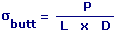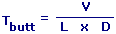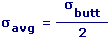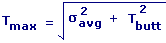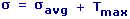P = Tensile Force L = Length Of Weld D = Throat Depth Of Weld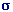butt = Normal Stress in Weld V = Shear Force Tbutt = Shear Stress in Weldavg = Average Stress in Weld Tmax = Maximum Shear Stress= Principal Stress
 Enter your values: Length Of Weld (L): Cm Throat Depth Of Weld (D): Cm Tensile Force (P): N Shear Force (V): N Results: Normal Stress in Weld: 106 N / m2 Shear Stress in Weld: 106 N / m2 Average Stress in Weld: 106 N / m2 Maximum Shear Stress: 106 N / m2 Principal Stress: 106 N / m2

 Web www.calculatoredge.com
 A welding technique used to join two tubes in which the squared and prepared ends are butted together in preparation for welding. The resulting circumferential weld has relatively good strength characteristics but has limitations where the tube is to be plastically deformed or bent, such as occurs on a coiled tubing string.

This calculator is used to calculate the resulting stresses acting in the weld.

Butt welds performed on a coiled tubing string should be checked carefully using hardness and radiographic testing methods and their locations detailed in the string record. The anticipated fatigue life in the butt-weld area must also be reduced to compensate for the weakness of the weld.

Stress is a measure of the average amount of force exerted per unit area. It is a measure of the intensity of the total internal forces acting within a body across imaginary internal surfaces, as a reaction to external applied forces and body forces.

Shear stress is a stress state where the stress is parallel or tangential to a face of the material, as opposed to normal stress when the stress is perpendicular to the face.

The magnitude of the normal stress component, of any stress vector acting on an arbitrary plane with normal vector at a given point in terms of the component of the stress tensor is the dot product of the stress vector and the normal vector.LINKSDISCLAIMERCONTACT US